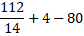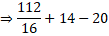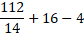Question of The Day13-01-2022

Which two numbers should be interchanged in the following equation to make it correct?

112 ÷ 14 + 16 – 5 × 4 = 1

Correct Answer : c ) 14 and 16

Explanation :

According to the question

Consider option (a)

4 and 14

Changing the digits

112 ÷ 4 + 16 – 5 × 14 = 1

⇒28 + 16 – 70

⇒ -26

⇒ -26 ≠1

Consider option (b)

16 and 4

Changing the digits

112 ÷ 14 + 4 – 5 × 16 = 1⇒ -68 ≠1

Consider option (c)

14 and 16

Changing the digits

112 ÷ 16 + 14 – 5 × 4 = 1⇒ 7 + 14 – 20

⇒ 1

⇒ 1 ≠ 1

Consider option (d)

1 and 5

Changing the digits

112 ÷ 14 + 16 – 1 × 4 = 5⇒8 + 16 – 4

⇒ 20

Hence, (c) is the correct answer.0